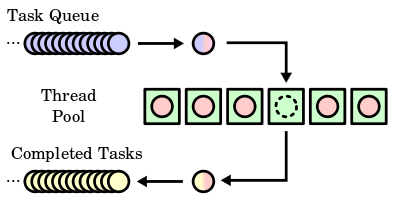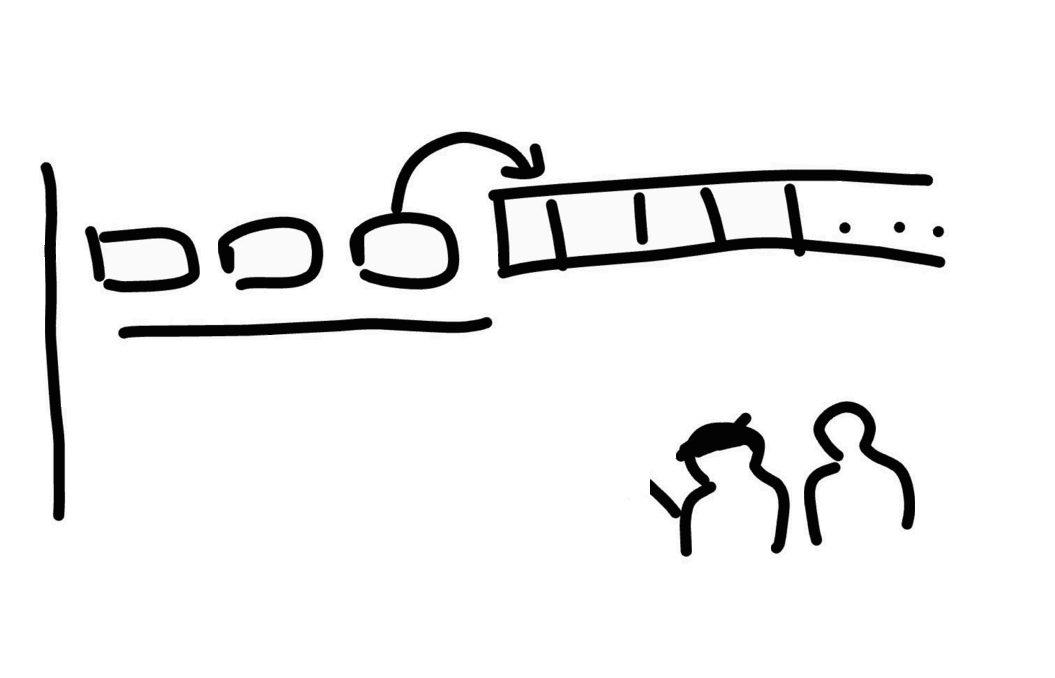Connecting...

# Purely functional parallelism in Scala by Wiem Zine El AbidineFind out how to how to implement purely functional design for parallelism with Software Engineer at MOIAWiem Zine El Abidine.

Wiem takes us through exactly what parallelism is and using a data structure execute parallel programs, so if you're looking for more functional programming knowledge you've got it here!

'This blog is about how to implement purely functional design for parallelism from “Functional programming in Scala” (red book)

We’ll go through that part step by step,

First of all I will introduce what is parallelism and how our parallel programs are executed in different Threads, then we’re going to design a data structure that can execute parallel programs using purely functional approach.

A computer reduces every task to a series of calculations and the CPU is the heart and the brain of the computer that carries out the instructions of our programs and of every computations.

Doing a lot of things at once saves time but we can’t just rely to do everything sequentially so we need to handle parallelism.The force of software comes from hardware that supports parallelism when computers provide multiple cores per CPU each capable of executing separate instructions.

Large problems can be divided into a smaller one (Tasks), which can be solved at the same time (in separate threads). This approach provides high performance (non blocking).

The traditional design of programs that run in parallel is to use: execution threads and shared mutable memory which leads to complexity because it interacts with different program parts that you cannot control very well and you have to make sure which value is putted in the mutable state before the other so the result could easily have race conditions, and that’s very hard to control, the code would be hard to reason about and hard to test..

Let’s think about how can we describe parallel Tasks (using the traditional design), and how could we improve it using data types and functions that enable the parallel computations!

We can think about using 'java.lang.Thread' and define our tasks as objects extends 'Runnable' and override 'run' to the computation, and when we start the task, our computation will be executed in separated thread.

``````trait Runnable { def run: Unit }
def start: Unit
def join: Unit
}``````

But:

1. Creating a Thread is an expensive operation

Example: if you have a CPU with 4 cores, maximum 4 threads can run at the same time. If you have more than the number of cores, some threads will be suspended until other threads finish their work.

In order to return the number of processors available to the JVM you can call:

``Runtime.getRuntime.availableProcessors()``The thread pool execution uses a blocking queue. It keeps storing all the tasks that you have submitted to the executior service, and all threads (workers) are always running and performing the same steps:

• Take the Task from the queue
• Execute it
• Take the next or wait until a task will be added to the queue

Example:

Assuming we have many Tasks (extends 'Runnable') and we create a thread pool with 2 workers.

``````val service: ExecutorService = Executors.newFixedThreadPool(2)
service.submit(t1)
service.submit(t2)
service.submit(t3)``````

Note: we can assume that our tasks will be executed in logical threads that will run concurrently with main execution thread of the program. You can see what I mean in this picture: the 2 painters are the threads (workers) they will execute the tasks, t1 and t3 are executed by the same thread but separately and t2 is executed by the other one.//TODO: we need to generalize our design to be useful for every type of executor Service.

2. 'Runnable' doesn’t return a meaningful value because 'run' returns 'Unit'. We cannot test the result of our parallel computations, we cannot control 'Runnable' because it’s hard to know about its internal behavior, so let’s think about a better approach to get the result of the computation after running the task.

We’re going to have an 'ExecutorService' and we will 'submit' our tasks to it, submit can take 'Runnable' or 'Callable[A]'

We can define our tasks as a 'Callable[A]' specifying the type of our computation result, when we 'submit' our tasks to the 'ExecutorService' they will be evaluated in a separate logical thread asynchronously because each of them returns a java 'Future[A]'

``````trait Callable[A] {
def call: A
}
class ExecutorService {
def submit[A](a: Callable[A]): Future[A]
}``````

2. Data type and functions

The data structure should be a container of a value that will be executed in separate thread and it requires an 'ExecutorService' to run the result and return it in the future, let’s call it 'Par':

``type Par[A] = ExecutorService => Future[A]``

In order to interpret 'Par' we need to define a method that requires an 'ExecutorService' and returns 'Future[A]'

``def run[A](es: ExecutorService)(par: Par[A]): Future[A] = par(es)``

Let’s start with a simple implementation that wraps a constant value, a function that creates a 'Par' of a single value that is always done and can’t be cancelled:

``````def unit[A](a: A): Par[A] = Par(_ => UnitFuture(a))
private case class UnitFuture[A](get: A) extends Future[A] {
def cancel(x: Boolean): Boolean = false
def get(v: Long, unit: java.util.concurrent.TimeUnit): A = get
def isCancelled(): Boolean = false
def isDone(): Boolean = true
}``````

Note, we’re using 'Future' which doesn’t have a purely functional interface.

We need to submit a computation that will run it in a logical thread:

``````def fork(a: => Par[A]): Par[A] = es =>
es.submit(new Callable[A] {
def call: A = run(es)(a).get
})``````

Here we see call by name: the 'a' will be evaluated only when it will be called (later in 'def call'.)

Let’s implement 'map2' to combine two parallel computations

``````def map2[A, B, C](a: Par[A], b: Par[B])(f: (A, B) => C): Par[C] =
(es: ExecutorService) => {
val af = run(es)(a)
val bf = run(es)(b)
UnitFuture(f(af.get, bf.get))
}``````

But this doesn’t evaluate the call of 'a' and 'b' in separate logical thread.

So we can fork 'a' and 'b' : 'map2(fork(a), fork(b))'

Let’s take a break and talk about other thing

In the red book, there is a section about The algebra of an API, it’s about how in FP it is important to have proof properties and laws about code. In general when you implement tests for the code, you try to break the expected behavior of the computation to reveal if there are bugs. In FP it’s quite similar but we have to do that during the implementation of the Algebra respecting some rules and strong FP properties. That’s why it’s easy to reason about a pure functional code.

There are two laws for the implementation of 'Par': the law of mapping, and the law of forking.

Let’s talk about that shortly, first of all we need the 'map' function here:

``````def map[A, B](a: Par[A])(f: A => B): Par[B] =
map2(a, unit(()))((a, _) => f(a))``````
• The law of mapping: identity and composition

'map(unit(x))(f) == map(unit(f(x)))' :There are proofs for those laws, you can read this interesting paper: free theorem

• The law of forking: 'fork(x) == x': which means 'fork' shouldn’t affect the result of the parallel computation.

We place constraints on what operation can mean. Making laws that seems reasonable and respecting them make us confident that there is no strange behaviors in our functions (like raising exception, or some other side effects).

So we use laws to test our implementation.

Let’s try to break the law of 'fork':

implement 'equals':

``````def equals[A, B](e: ExecutorService)(p1: Par[A], p2: Par[B]): Boolean =
run(e)(p1).get == run(e)(p2).get``````

Add a 'lazyUnit' and check the law of 'fork' using 'equals'

``````def lazyUnit[A](a: => A): Par[A] = fork(unit(a))

equals(es)(fork(lazyUnit(1)), lazyUnit(1))``````

And run it using an 'ExecutorService' backed by a 'FixedThreadPool' with max size: 1. This results in this code a deadlocking.

This will block the program because in 'fork' when we’re submitting the 'Callable', 'run' will submit another 'Callable' to the 'ExecutorService' and get back the 'Future' but there are no threads available to run this 'Callable'

This tells us that we need either to improve the implementation or we can refine the law a bit and use an unbounded thread pool.

Let’s think about a solution to improve the implementation of 'fork' with fixed number of threads:

Non blocking Par

The problem with the current implementation is that 'Future.get' will block the current thread in order to get the value out.

Let’s think about on implementing our own version of 'Future' (following the magic red book recipe) that can register a callback that will be invoked when the result is ready:

``````sealed trait Future[A] {
private[parallelism] def apply(k: A => Unit): Unit
}``````

instead of 'get' we have a private (outside the package) 'apply' that has a callback: 'k

Using local side effect in a pure API

“The 'Future' type we defined here is rather imperative. An 'A => Unit?' Such a function can only be useful for executing some side effect using the given 'A', as we certainly aren’t using the returned result. Are we still doing functional programming in using a type like 'Future'? Yes, but we’re making use of a common technique of using side effects as an implementation detail for a purely functional API. We can get away with this because the side effects we use are not observable to code that uses Par. Note that 'Future.apply' is protected and can’t even be called by outside code.” Functional Programming in Scala — p:116

Now the implementation of 'unit' using 'Future':

``````def unit[A](a: A): Par[A] = (_: ExecutorService) => new Future[A] {
def apply(cb: A => Unit): Unit = cb(a)
}``````

How could we implement 'fork' now?

``````def fork[A](a: => Par[A]): Par[A] =
es =>
new Future[A] {
def apply(cb: A => Unit): Unit =
es.submit(new Callable[Unit] { def call = a(es)(cb) })
}``````

But wait, how could we get back the computation result of type 'A'?

The callback in 'Future' has an input of type 'A' this is our suspended computation that will wait until it will be invoked, when it will be invoker? in the 'run' method.

The tricky implementation of 'run':

'run' will block the current thread until the result of type 'A' will be available

``def run[A](es: ExecutorService)(p: Par[A]): A = ???``

So the users should call 'run' only when they definitely want to wait for the result let’s call it 'unsafeRun'

``````def unsafeRun[A](es: ExecutorService)(p: Par[A]): A = {
val ref = new AtomicReference[A]
val latch = new CountDownLatch(1)
p(es) { a =>
ref.set(a)
latch.countDown()
}
ref.get
}``````
• 'AtomicReference': is a thread safe reference to store the result.
• 'CountDownLatch': allows threads to wait until its 'countDown' method is called a certain number of times.

So once we receive the value of 'a' from 'p', we store it in 'ref' and at the end we return it from 'ref'.

In order to implement 'map2' we need to run both 'Par' in parallel, when we receive both results we need to invoke 'f' and then pass the resulting value of type 'C' to the callback of 'Future.apply'. There are several race conditions to worry about.

There is a tricky implementation of 'NonBlockingPar' here that uses 'Actor' with a minimized implementation, here.

Conclusion

In our programming daily life we have used asynchronous and concurrent programming libraries to provide high performance for our parallel computations, in this blog we covered the concept of parallelism and how can we design a purely functional parallelism API.

As you see if we didn’t define laws to our API we wouldn’t discover the thread resource leak in the first representation and during writing code, we figured out which way makes more sense.

Every situation that you’ll encounter makes you change your way, don’t stop improving, the elegant solution will not come to mind right away, it’s cool to find a better solution several times. Don’t stop improving, keep going.

I hope that you’ve enjoyed reading this blog.'

This article was written by Wiem Zine El Abidine and posted originally on Medium